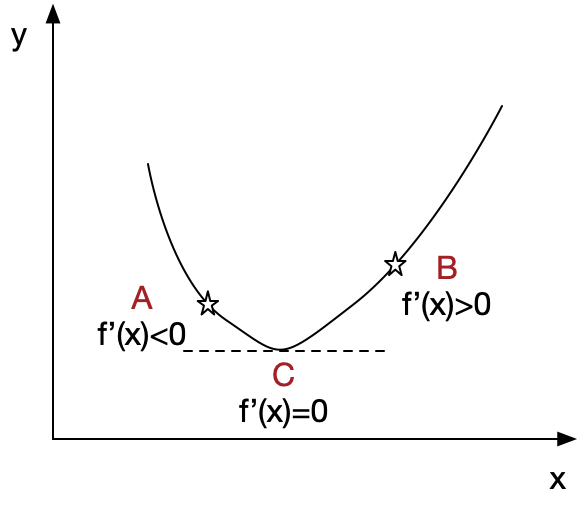Stochastic Gradient decent is one of the fundamental algorithm in deep learning. It is used when we perform optimization of the cost function.Suppose the function is $f(x)$As what illustrated above, we want to approach the minimum value of f(x) which is the point C. If we are now at point A, the derivation of A is f’(x)>0, so we need to go right down which is the opposite direction of f’(x). If we stand at B,the derivation of A is f’(x)>0, we should go left down which is also the opposite direction of f’(x).

According to the optimization strategy , we should update the parameter like this: $$x = x - \eta f’(x)$$ $\eta$ is so-called the learning rate, it determines the size of steps we take to reach a minimum value of $f(x)$

Follow the rules of gradient descent, when we perform one update of parameters the intuitive strategy is to calculate the gradients for all the examples in the dataset and sum them up, this computation process is also called batch gradient.

The pseudocode described above looks like this.

for i in range(epoches):
param = param - learning_rate * params_grad


For each update we need to walk through all these examples, if there are millions of them, the computation cost would be a problem, let alone there usually are several epochs of updates.

The inefficiency of the batch gradient leads to another strategy that is widely used in deep learning, which is stochastic gradient descent(SGD).

Instead of updating the params based on the gradients of all examples, SGD updates the param after computing the gradient of one example, what particular is the dataset should be shuffled in each epoch.

The poseudocode of SGC looks like this:

for i in range(epochs):
np.random.shuffle(data)
for example in data:

for i in range(epochs):